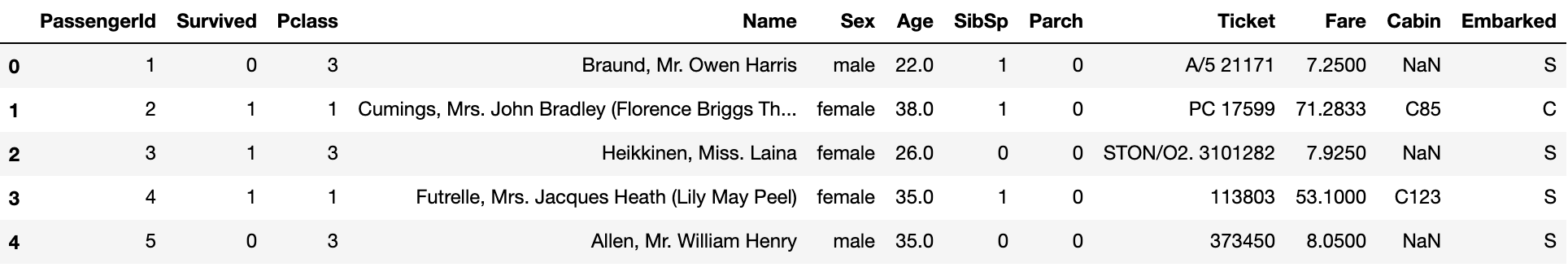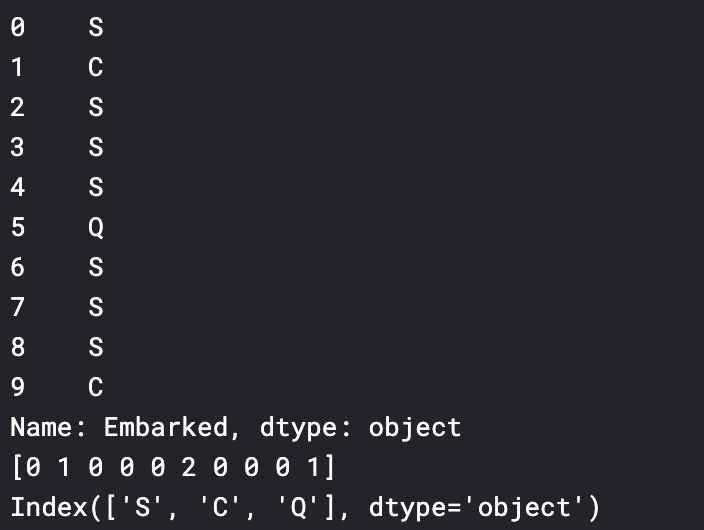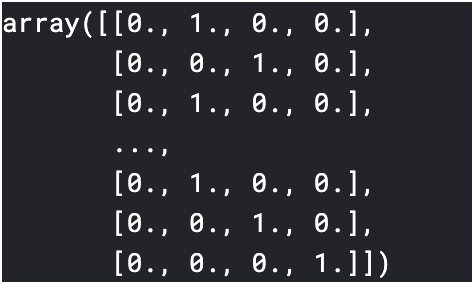# “Kaggle Memorandum” Conversion to One-hot Vector

## Purpose

Convert qualitative variables (categorical variables) to One-hot vectors

## Usage data / environment

Data: Kaggle’s Titanic data

Environment: kaggle notebook

## Method

#### `onehot_encoding.py`

``````
#Module import, os preparation
import numpy as np
import pandas as pd
import matplotlib as plt
import os
for dirname, _, filenames in os.walk('/kaggle/input'):
for filename in filenames:
print(os.path.join(dirname, filename))
``````

#### `onehot_encoding.py`

``````
``````

Take a look at the data

#### `onehot_encoding.py`

``````
``````You can see that there are some data frames of categorical variables. We aim to convert these into One-hot vectors.

For the time being, it is difficult to handle the character string as it is, so assign different numerical values to each category.
Use Pandas’s `factorize ()`.

`factorize ()` returns both numeric data (emb_cat_encoded) and a list of categories (emb_categories).

#### `onehot_encoding.py`

``````
train_cat=train_data['Embarked']
train_cat_encoded,train_categories=train_cat.factorize()

#Take a look
print(train_cat_encoded[:10])
print(train_categories)
``````Then convert to one-hot vector

Use OneHotEncoder provided by scikit-learn.

#### `onehot_encoding.py`

``````
#scikit-Import OneHotEncoder from learn
from sklearn.preprocessing import OneHotEncoder

#one-Convert to hot vector
oe=OneHotEncoder(categories="auto")
train_cat_1hot=oe.fit_transform(train_cat_encoded.reshape(-1,1))

#Take a look inside
train_cat_1hot
``````Conversion completed.